Smartick is a fun way to learn math!I want to learn about: Operations & Algebraic Thinking

Learn operations and algebraic thinking in elementary with Smartick. Exercises, theory, tutorials, and teaching resources about operations and algebraic thinking in elementary aged children. Here you will find posts about operations and algebraic thinking to make learning mathematics easier and more fun.

Jul23

Mental Math Strategies for Children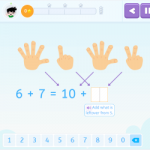Scientific evidence tells us that children with learning difficulties who do not use mental math strategies have poor results in this area, similar to those of children with learning difficulties specific to math. That is why at Smartick we wanted to make sure that all children develop mental math strategies. In this post, we are […]

Apr21

Subtraction With and Without CarryingToday we are going to learn how to solve subtraction problems both with and without carrying. We will begin with a review of what subtraction is and its elements, and then we will see an example of each type of subtraction with a video. What is Subtraction? Subtraction is a mathematical operation that consists of […]

Apr13

What is Multiplication: Steps to Learn and Understand Multiplication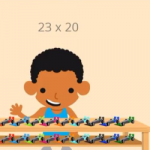What is multiplication? We will explain it in this post! Then we will show some examples using the steps that you should follow to complete multiplication problems, along with a video. What is Multiplication? Multiplication is a mathematical operation that is performed to calculate the result by adding the number as many times as indicated […]

May04

Incomplete Horizontal Addition and Subtraction to Develop Mathematical Flexibility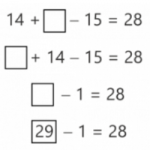Today we are going to solve incomplete horizontal addition and subtraction exercises by using different strategies. In mathematics, it is important to know how to use different strategies for solving the same problem or exercise. Knowing various strategies, how to apply them, and knowing when is the best time to use each, is what we […]

Apr06

The Reason for Divisibility CriteriaSometimes we may be interested in knowing if one number is divisible by another, without having to perform a division. This is especially useful when we work with large numbers that require a lot of time for completing calculations. If you would like to refresh your memory on divisibility criteria, take a look at this […]

Mar09

Reducible Equations: What Are They? How Do We Solve Them?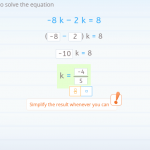In this post, we are going to explain reducible equations with several examples taken from Smartick’s daily sessions. We will begin with some easier examples, and work our way toward those that are more complicated. If you need to review what an equation is, and its terms, you can take a look at this previous […]

Feb03

Working on Combined Operations through Word ProblemsIn today’s post, we are going to present the way that we work on combined operations through word problems in Smartick. In the field of mathematics in school, combined operations are those problems where we need to use more than one operation. If you don’t remember exactly what they were, click here to refresh your memory! […]

Nov11

Multiplication of Decimals Using MoneyToday we are going to better understand the multiplication of decimals by using a very common manipulative material: money. It is going to help us understand why multiplying by 10 moves the decimal position to the right. Equivalence of Bills or Coins with Numbers First, we are going to establish the equivalence of each coin […]

Sep30

The Associative Property and Mental Math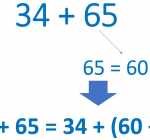In today’s post, we will see how useful the associative property can be when we want to do mental math with addition and multiplication. To begin, I would recommend that you review these earlier posts from our blog about the associative property that could be very useful: Addition and Multiplication Multiplication What is the associative […]

Sep09

Alternative Methods for Multiplication: Russian and Hindu Methods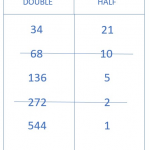In today’s post, we are going to learn two methods to solve multiplication problems that are different from the ones we are used to using. First, we are going to travel to Russia where we will learn how to multiply using the Russian Method. Then, we will continue on to India to learn the Hindu […]

Sep02

What is Factorial Decomposition?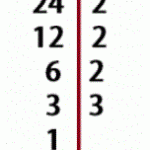In today’s post we are going to answer the question, what is factorial decomposition? I hope that by the end of the post you’ll have it crystal clear. Definition of Factorial Decomposition We are going to start by remembering what a ”factor” is in mathematics. A factor is every number that is multiplied by another. […]